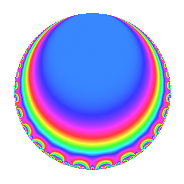# Properties

 Label 148.1.fLevel 148 Weight 1 Character orbit f Rep. character $$\chi_{148}(105,\cdot)$$ Character field $$\Q(\zeta_{4})$$ Dimension 2 Newforms 1 Sturm bound 19 Trace bound 0

# Related objects

## Defining parameters

 Level: $$N$$ = $$148 = 2^{2} \cdot 37$$ Weight: $$k$$ = $$1$$ Character orbit: $$[\chi]$$ = 148.f (of order $$4$$ and degree $$2$$) Character conductor: $$\operatorname{cond}(\chi)$$ = $$37$$ Character field: $$\Q(i)$$ Newforms: $$1$$ Sturm bound: $$19$$ Trace bound: $$0$$

## Dimensions

The following table gives the dimensions of various subspaces of $$M_{1}(148, [\chi])$$.

Total New Old
Modular forms 8 2 6
Cusp forms 2 2 0
Eisenstein series 6 0 6

The following table gives the dimensions of subspaces with specified projective image type.

$$D_n$$ $$A_4$$ $$S_4$$ $$A_5$$
Dimension 0 0 2 0

## Trace form

 $$2q - 2q^{7} + O(q^{10})$$ $$2q - 2q^{7} - 2q^{17} + 2q^{19} - 2q^{23} - 2q^{29} + 2q^{33} + 2q^{47} + 2q^{51} + 2q^{53} - 2q^{57} + 2q^{69} + 2q^{71} + 2q^{75} - 2q^{79} - 2q^{81} - 2q^{83} - 2q^{87} - 2q^{89} + O(q^{100})$$

## Decomposition of $$S_{1}^{\mathrm{new}}(148, [\chi])$$ into irreducible Hecke orbits

Label Dim. $$A$$ Field Image CM RM Traces $q$-expansion
$$a_2$$ $$a_3$$ $$a_5$$ $$a_7$$
148.1.f.a $$2$$ $$0.074$$ $$\Q(\sqrt{-1})$$ $$S_{4}$$ None None $$0$$ $$0$$ $$0$$ $$-2$$ $$q+iq^{3}-q^{7}-iq^{11}+(-1-i)q^{17}+\cdots$$